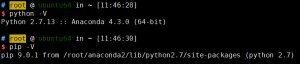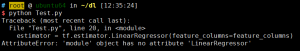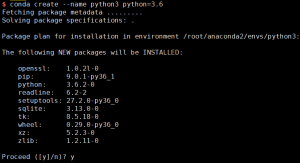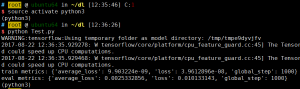# 深度学习之TensorFlow环境搭建

5412℃

TensorFlow支持Ubuntu、Mac OS X、Windows，也支持从源码安装。本文以Ubuntu操作系统为例，来讲解TensorFlow的环境搭建过程。

### TensorFlow分为两个版本：

• CPU支持版本如果系统没有安装GPU，那么只能使用TensorFlow的CPU支持版本。
• GPU支持版本如果系统安装了GPU，并且添加了其他相关支持(安装CUDA Toolkit、cuDNN、libcupti-dev库等)，则可以使用TensorFlow的GPU支持版本。

• virtualenv安装
• 原生的pip安装
• Docker安装
• Anaconda安装
• 从源码安装

### 在Ubuntu上安装TensorFlow

``````
\$ cat /etc/lsb-release
DISTRIB_ID=Ubuntu
DISTRIB_RELEASE=14.04
DISTRIB_CODENAME=trusty
DISTRIB_DESCRIPTION="Ubuntu 14.04.5 LTS"
````````````
pip install tensorflow
``````

``````
>>> import tensorflow as tf
>>> sess = tf.Session()
>>> hello = tf.constant('hello world!')
>>> print(sess.run(hello))
hello world!
``````

### Python2与Python3的版本切换

``````
import numpy as np
import tensorflow as tf

'''
tf.estimator
'''

x_train = np.array([1., 2., 3., 4.])
y_train = np.array([0., -1., -2., -3.])
x_eval = np.array([2., 5., 8., 1.])
y_eval = np.array([-1.01, -4.1, -7, 0.])
input_fn = tf.estimator.inputs.numpy_input_fn(
{"x": x_train}, y_train, batch_size=4, num_epochs=None, shuffle=True)
train_input_fn = tf.estimator.inputs.numpy_input_fn(
{"x": x_train}, y_train, batch_size=4, num_epochs=1000, shuffle=False)
eval_input_fn = tf.estimator.inputs.numpy_input_fn(
{"x": x_eval}, y_eval, batch_size=4, num_epochs=1000, shuffle=False)

feature_columns = [tf.feature_column.numeric_column("x", shape=)]
estimator = tf.estimator.LinearRegressor(feature_columns=feature_columns)

estimator.train(input_fn=input_fn, steps=1000)

train_metrics = estimator.evaluate(input_fn=train_input_fn)
eval_metrics = estimator.evaluate(input_fn=eval_input_fn)
print("train metrics: %r"% train_metrics)
print("eval metrics: %r"% eval_metrics)
``````#### 搭建Python3环境：#### 激活python3环境，并运行Test.py：